# Samacheer Kalvi 10th Maths Book Solutions Chapter 6 | Trigonometry Answers For Tamil Nadu Board

Samacheer Kalvi 10th Maths Book Solutions Chapter 6 – Trigonometry is available here. The Samacheer Kalvi 10th Maths book answers of Chapter 6, available at BYJU’S, contain step by step explanations designed by our Mathematics experts. All these important questions are based on the new pattern prescribed by the Tamil Nadu board. Students can also get the solutions of other chapters on Samacheer Kalvi 10th Maths solutions. Solving the Samacheer Kalvi Class 10 Maths Textbook Chapter 6 questions first and then referrring to the Solutions is the best way to gauge the performance. This will help the students to plan their studies and then perform well to the board exams.

Chapter 6 of the Samacheer Kalvi 10th Maths guide will help the students to solve problems related to trigonometric identities, heights and distances, problems involving angle of elevation and depression.

### Samacheer Kalvi 10th Maths Chapter 6: Trigonometry Book Exercise 6.1 Questions and Solutions

Question 1: Prove the following identity: cos θ / (1 + sin θ) = sec θ – tan θ.

Solution:

cos θ / (1 + sin θ)

By multiplying the conjugate of denominator,

= [cos θ / (1 + sin θ)] [(1 – sin θ) / (1 – sin θ)]

= [cos θ (1 – sin θ) / (1 – sin θ) (1 + sin θ)]

= [cos θ (1 – sin θ) / (1 – sin 2θ)]

= [cos θ(1 – sin θ) / cos 2θ]

= (1 – sin θ) / cos θ

= (1 / cos θ) – (sin θ / cos θ)

= sec θ – tan θ

Question 2: Prove the following identity: sec6θ = tan6θ + 3 tan2θ sec2θ + 1

Solution:

RHS :

tan6θ + 3 tan2θ sec2θ + 1

= (tan2θ)3 + 3 (tan2θ) (sec2θ) + 1

(a + b)3 = a3 + b3 + 3ab (a + b)

= (tan2θ)3 + 3 (tan2θ) 1 (tan2θ + 1) + 13

= (tan2θ + 1)3

= (sec2θ)3

= sec6θ

Question 3: If sinθ + cosθ = p and secθ + cosecθ = q, then prove that q(p2 −1) = 2p

Solution:

p = sinθ + cosθ

p2 = (sinθ + cosθ)2

p2 = sin2θ + cos2θ + 2sinθ cosθ

p2 = 1 + 2sinθ cosθ

p2 – 1 = 1 + 2sinθ cosθ – 1

p2 – 1 = 2sinθcosθ

q(p2 −1) = (secθ + cosecθ) (2sinθcosθ)

q(p2 −1) = (1 / cosθ) + (1 / sinθ) (2sinθcosθ)

q(p2 −1) = [(2sinθcosθ) / cosθ] + [(2sinθcosθ) / sinθ]

q(p2 −1) = 2sinθ + 2cosθ

q(p2 −1) = 2(sinθ + cosθ)

q(p2 −1) = 2p

Question 4: If sinθ(1 + sin2θ) = cos2θ, then prove that cos6θ – 4cos4θ + 8cos2θ = 4

Solution:

sinθ(1 + sin2θ) = cos2θ

sinθ + sin3θ = 1 – sin2θ

sinθ + sin2θ + sin3θ = 1

sinθ + sin3θ = 1 – sin2θ

Square both sides,

(sinθ + sin3θ)2 = (1 – sin2θ)2

sin2θ + 2sin4θ + sin6θ = 1 – 2sin2θ + sin4θ

sin2θ + 2sin2θ + 2sin4θ – sin4θ + sin6θ = 1

3sin2θ + sin4θ + sin6θ = 1

3(1 – cos2θ) + (1 – cos2θ)2 + (1 – cos2θ)3 = 1

3 – 3cos2θ + 1 + cos4θ – 2cos2θ + 1 – 3cos2θ + 3cos4θ – cos6θ = 1

4 – cos6θ + 4cos4θ – 8cos2θ = 0

cos6θ − 4cos4θ + 8cos2θ = 4

### Samacheer Kalvi 10th Maths Chapter 6: Trigonometry Book Exercise 6.2 Questions and Solutions

Question 1: A road is flanked on either side by continuous rows of houses of height 4√3 m with no space in between them. A pedestrian is standing on the median of the road facing a row house. The angle of elevation from the pedestrian to the top of the house is 30°. Find the width of the road.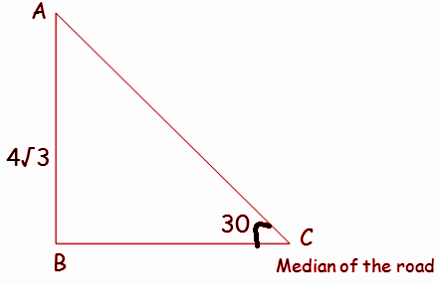Solution:

AB = opposite side, BC = adjacent side, AC = hypotenuse side

tan θ = opposite side / adjacent side

tan 30 = AB / BC

1 / √3 = 4√3 / distance from the median of the road to the house

BC = 4√3(√3) = 12

Required distance = 2 * BC = 2 (12) = 24 m

Question 2: To a man standing outside his house, the angles of elevation of the top and bottom of a window are 60° and 45° respectively. If the height of the man is 180 cm and if he is 5 m away from the wall, what is the height of the window? (√3 = 1.732)

Solution: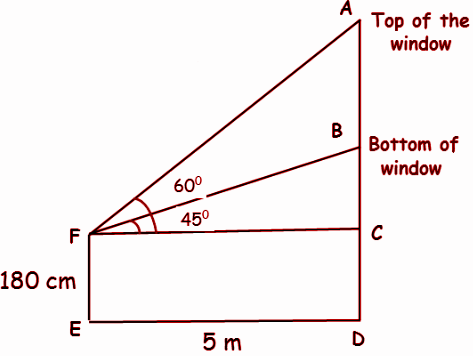Height of window = AB

180 cm = 1.8 m

In △FBC,

tan θ = opposite side / adjacent side

tan 45 = BC / FC

1 = BC / 5

BC = 5 m

In △FBC,

tan 60 = AC / FC

√3 = AC / 5

AC = 5√3

AB = AC – BC

= 5√3 – 5

= 5(√3 – 1)

= 5(1.732 – 1)

= 5(0.732)

= 3.66 m

Hence, the height of the window is 3.66 m.

Question 3: A vertical pole fixed to the ground is divided in the ratio 1:9 by a mark on it with the lower part shorter than the upper part. If the two parts subtend equal angles at a place on the ground, 25 m away from the base of the pole, what is the height of the pole?

Solution: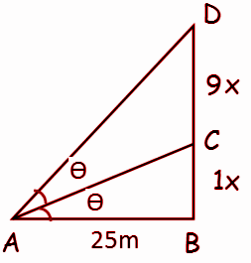By using the angle bisector theorem,

Let the two parts subtend equal angles at point A such that

CAB = DAC = θ

BC / DC = AB / AD

1 / 9 = 25 / AD

2252 = 252 + (10x)2

100x2 = 2252 – 252

100x2 = (225 + 25) (225 – 25)

100x2 = (250) (200)

x2 = 500

x = 100√5 m

Question 4: A traveller approaches a mountain on the highway. He measures the angle of elevation to the peak at each milestone. At two consecutive milestones, the angles measured are 4° and 8°. What is the height of the peak if the distance between consecutive milestones is 1 mile? (tan 4° = 0.0699, tan 8° = 0.1405)

Solution: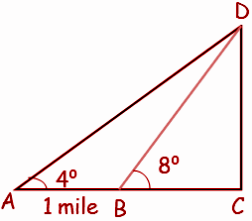Let BC = x

In △BDC,

tan 8 = DC / BC

0.1405 = DC / x

DC = 0.1405 x —- (1)

tan 4 = DC / AC

0.0699 = DC / (1 + x)

DC = 0.0699(1 + x) —-(2)

(1) = (2)

0.1405 x = 0.0699(1 + x)

0.1405 x = 0.0699 + 0.0699 x

(0.1405 – 0.0699)x = 0.0699

0.0706 x = 0.0699

x = 0.0699 / 0.0706

x = 699 / 706

x = 0.99

By applying the value of x in (1), we get

DC = 0.1405 (0.99)

DC = 0.14 mile

So, the height of the peak is 0.14 mile.

### Samacheer Kalvi 10th Maths Chapter 6: Trigonometry Book Exercise 6.3 Questions and Solutions

Question 1: From the top of the tower 60 m high the angles of depression of the top and bottom of a vertical lamp post are observed to be 38° and 60°, respectively. Find the height of the lamp post. (tan 38° = 0.7813, √3 = 1.732)

Solution: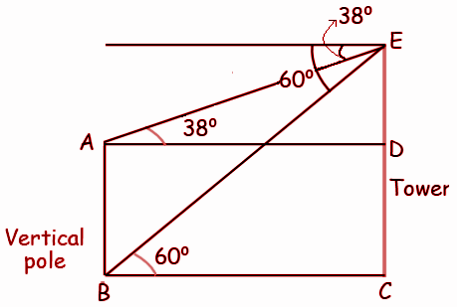Height of tower = 60 m

Let DC = x, ED = 60 – x

tan 38 = DE / AD

0.7813 = (60 – x) / AD

AD = (60 – x) / 0.7813 —(1)

In △BEC,

tan 60 = EC / BC

√3 = 60 / BC

BC = 60 / √3

BC = 20√3 ——(2)

(1) = (2)

(60 – x) / 0.7813 = 20√3

60 – x = 20 (0.7813) (1.732)

60 – x = 34.64(0.7813)

60 – x = 27.06

x = 60 – 27.06

x = 32.94

So, the height of the vertical lamp post is 32.94 m

Question 2: An aeroplane at an altitude of 1800 m finds that two boats are sailing towards it in the same direction. The angles of depression of the boats as observed from the aeroplane are 60° and 30° respectively. Find the distance between the two boats. (√3 = 1.732)

Solution: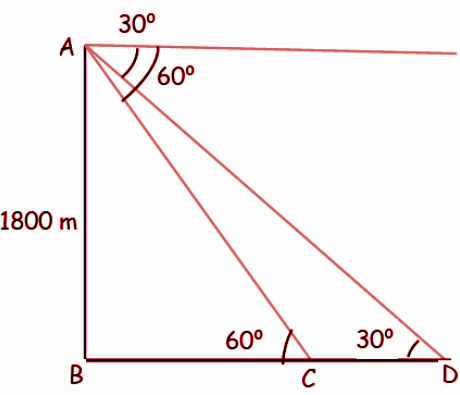In △ABC,

tan θ = opposite side / adjacent side

tan 60 = AB / BC

√3 = 1800 / BC

BC = 1800 / √3

BC = 600√3

In △ABD,

tan 30 = AB / BD

1 / √3 = 1800 / BD

BD = 1800√3

Distance between two boats = CD

= BD – BC

= 1800√3 – 600√3

= 1200 √3

= 1200(1.732)

Distance between two boats = 2078.4 m

Question 3: From the top of a lighthouse, the angle of depression of two ships on opposite sides of it is observed to be 30° and 60°. If the height of the lighthouse is h meters and the line joining the ships passes through the foot of the lighthouse, show that the distance between the ships is 4h / √3 m.

Solution: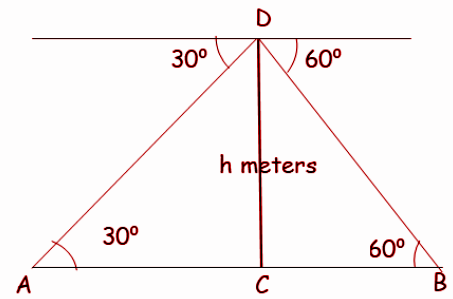tan 30 = DC / AC

1 / √3 = h / AC

AC = √3 h —–(1)

In △DCB,

tan 60 = DC / BC

√3 = h / BC

BC = h / √3 —–(2)

(1) + (2)

AC + BC = √3 h + (h / √3)

Distance between two ships = (3h + h) / √3

Distance between two ships = 4h / √3

Question 4: A lift in a building of height 90 feet with transparent glass walls is descending from the top of the building. At the top of the building, the angle of depression to a fountain in the garden is 60°. Two minutes later, the angle of depression reduces to 30°. If the fountain is 30√3 feet from the entrance of the lift, find the speed in ft/sec of the lift which is descending.

Solution: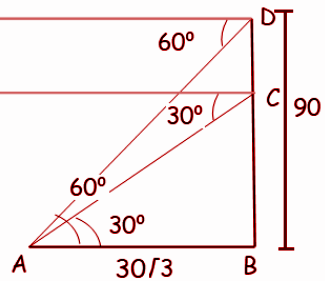In the diagram above, in the right triangle ABC,

tan 30° = opposite side / adjacent side

1 / √3 = BC / 30√3

30 = BC

DC = DB – CB

DC = 90 – 30

DC = 60 feet

So, the left has descended 60 ft in 2 minutes.

Speed = Distance / Time

= 60 / 2

= 30 ft / min

= 30 ft / (60 seconds)

= 30 / 60 ft /sec

= 0.5 ft / sec

So, the speed of the lift which is descending is 0.5 ft / sec.

### Samacheer Kalvi 10th Maths Chapter 6: Trigonometry Book Exercise 6.4 Questions and Solutions

Question 1: From the top of a tree of height 13 m the angle of elevation and depression of the top and bottom of another tree are 45° and 30°, respectively. Find the height of the second tree. (√3 = 1.732)

Solution: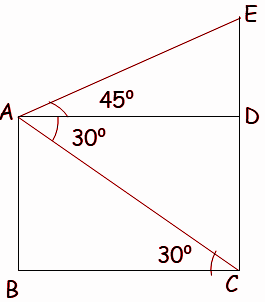AB = 13 m = DC

In △AED,

tan 45 = ED / AD

In △ABC,

tan 30 = AB / BC

1 / √3 = 13 / BC

BC = 13√3 —- (2)

By applying the value of AD in (1),

ED = 13√3

The height of second tree = ED + DC

= 13√3 + 13

= 13 (√3 + 1)

= 13 (1.732 + 1)

= 13 (2.732)

= 35.516 m

Hence, the height of the second tree is 35.52 m.

Question 2: A man is standing on the deck of a ship, which is 40 m above water level. He observes the angle of elevation of the top of a hill as 60° and the angle of depression of the base of the hill as 30°. Calculate the distance of the hill from the ship and the height of the hill. (√3 = 1.732)

Solution: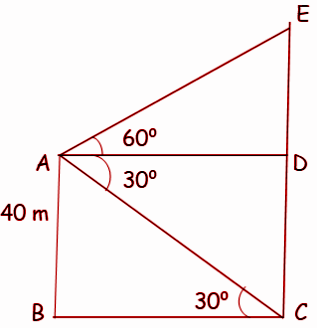In △AED,

tan 60 = ED / AD

AD = ED / √3 —– (1)

In △ABC,

tan 30 = AB / BC

1 / √3 = 40 / BC

BC = 40√3 —– (2)

(1) = (2)

40√3 = ED / √3

ED = 40√3 (√3) = 40(3)

ED = 120 m

Height of hill = ED + DC

= 120 + 40

= 160 m

Distance from hill to ship = 40√3

= 40(1.732)

= 69.28 m

Question 3: If the angle of elevation of a cloud from a point ‘h’ meters above a lake is θ1 and the angle of depression of its reflection in the lake is θ2. Prove that the height that the cloud is located from the ground is h (tan θ1+ tan θ2) / (tan θ2 – tan θ1).

Solution: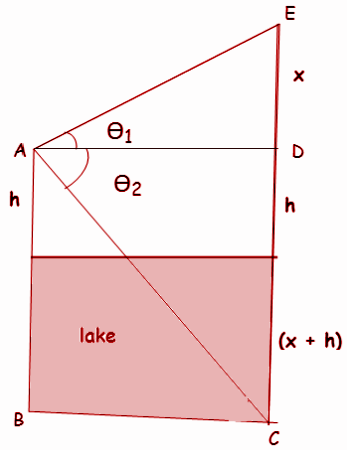In △AED,

tan θ1 = ED / AD

AD = x / tan θ1

AD = x cot θ1 —–(1)

tan θ2 = DC / AD

tan θ2 = (2h + x) / AD

AD = (2h + x) / tan θ2

AD = (2h + x) cot θ2 —– (2)

(1) = (2)

x cot θ1 = (2h + x) cot θ2

x cot θ1 = 2h cot θ2 + x cot θ2

x (cot θ1 – cot θ2) = 2h cot θ2

x (tan θ2 – tan θ1) / (tan θ1 tan θ2) = 2h (1 / tan θ2)

2h = [x tan θ2 (tan θ2 – tan θ1)] / (tan θ1 tan θ2)

2h = x(tan θ2 – tan θ1) / tan θ1

x = 2h tan θ1 / (tan θ2 – tan θ1)

Height of cloud = x + h = [2h tan θ1 / (tan θ2 – tan θ1)] + h

= [2h tan θ1+ h(tan θ2 – tan θ1)] / (tan θ2 – tan θ1)]

= h [tan θ1+ tan θ2] / (tan θ2 – tan θ1)]

Question 4: The angle of elevation of the top of a cell phone tower from the foot of a high apartment is 60° and the angle of depression of the foot of the tower from the top of the apartment is 30°. If the height of the apartment is 50 m, find the height of the cell phone tower. According to radiations control norms, the minimum height of a cell phone tower should be 120 m. State if the height of the above-mentioned cell phone tower meets the radiation norms.

Solution: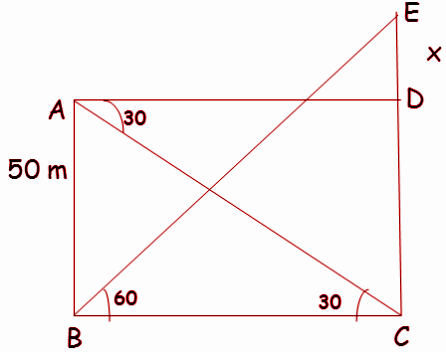In △BEC,

tan θ = opposite side / adjacent side

tan 60 = EC / BC

√3 = (50 + x) / BC

BC = (50 + x) / √3 —-(1)

In △ABD,

tan 30 = AB / BC

1/√3 = 50 / BC

BC = 50√3 —-(2)

(1) = (2)

(50 + x) / √3 = 50√3

50 + x = 50√3√3

50 + x = 150

x = 150 – 50

x = 100

Height of cell phone tower = 100 + 50 = 150 m

Hence, the cell phone tower meets the radiation norms.